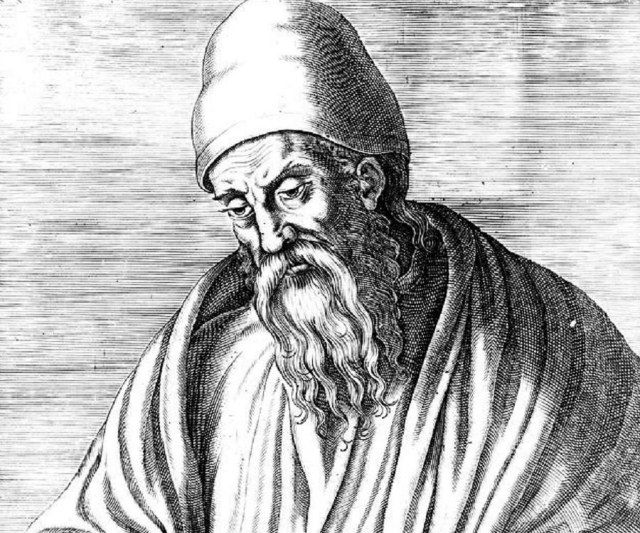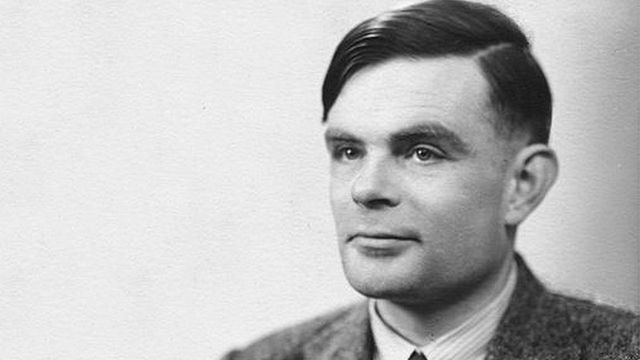# The Origin and Evolution of Algorithms

Souvik Das | Published 03 May 2016• ## From Babylonians to today's cryptographers, algorithms have progressed meticulously, keeping the essence of operations somewhat intact.

Origins is a monthly column, where we will be talking about the history of various innovative technologies that we take for granted today.

By definition, an algorithm is a pre-defined, self-contained set of instructions required to execute diverse functions. Such rules date back to as far as 300 BC, found inscribed on Babylonian clay tablets. The very basic algorithms were marking schemes that the ancient folks used to keep track of their grain stock and cattle. This was followed by the advent of the numeric system, and a subsequent evolution of abacus, algebra and variables followed, giving rise to symbols and rules involved in formulating evaluation systems.

Algorithms find their place in computer programs and mechanical applications. The origin of the term is attributed to Persian astronomer and mathematician, Abu Abdullah Muhammad ibn Musa Al-Khwarizmi (c. 850 AD). His works included introducing the decimal positioning within numeric systems to the Western world, along with the first ever systematic solution of linear and quadratic equations. In its original, rudimentary form, algorithms, known as algorism, was regarded as rules for computing calculations and performing arithmetic with Hindu-Arabic numerals. Later, with the Latin translation of Al-Khwarizmi’s name, algorisms set definite standards for performing computations to execute tasks.

Algorithms as we know today were only put into place with the advent and rise of mechanical engineering and processes. In its original form, algorithms gave base to the algebra of logic, using variables in calculations. The earliest instances of algorithms include Euclid’s function of greatest common divisor in numerics, Archimedes’ approximation of Pi, and Eratosthenes’ calculation of prime numbers. However, the first person to use the initial form of the term algorithm was 12th century English philosopher, Adelard de Bath, who used the term algorismus when translating Al-Khwarizmi’s Arabic works.Euclid: The foundation stone for possibly the world first functional algorithm

A major series of achievements in the evolution of algorithms came during the 1800s, the first of which was established by English mathematician George Boole, who also penned The Laws of Thought and established Boolean Algebra. In 1847, Boole unified logic with calculations and formed binary algebra, the basis of computing logic of today. In 1888, Giuseppe Peano established the axiomatization of mathematics (an axiom is an empirical rule established in certain fields, and used without exceptions universally). He used equations with symbols to obtain results. These would later go on to become rules that modern day mathematics and algorithms are based on.

Decades later, algorithms of the present form came into being with Alan Turing’s computing machine. Alongside, Alonzo Church’s Lambda Calculus became the calculating equivalent of Turing Machines, wherein a variable was bound to specific functions or executable equations to carry out operations. Turing’s computer model was based on the notion of a human computer and “states of mind” or environments, in which functions written down in symbols would find application. This was further broken down into basic squares, with each contributing to a specific operation. Each of these basic squares would hold a symbol, and each symbol would be finite in its own state and relativity.

A computer’s action would depend upon the tally of logic - each of these symbols acting as bits of data, and the computer’s “state of mind” being the condition or purpose of the function being executed. Turing also imposed a limit to the number of symbols being tallied at the same time, and computing more complex logic would require the machine to execute similar, consecutive operations. Each of these operations, hence, would be the simplest unit within the executable ‘code’. A code would be the entire compilation of the sets of functions, or the algorithm, based on which a problem would be solved.

"We may now construct a machine to do the work of this computer"

Turing’s logic of algorithms also laid down the foundation for operations involving variable factors, wherein a subsequent symbol would be altered based on the computation between two preceding symbols. In simpler words, when a function between two variables are executed, the resultant would be the figure that would be used in computing with the next variable, thereby giving rise to progressive algorithms that take into account changing values to compute a code. If need be, these operations would also define the outcome, altering the “state of mind” or parameters of a calculation. “We may now construct a machine to do the work of this computer,” Turing deduced in his theory.

Meanwhile, the foundation laid by Alan Turing, and also applicable to Church’s Lambda Calculus (stated earlier), was worked upon by Church’s student and American logician, John Barkley Rosser. Defining an “effective mathematical method”, Rosser stated that each step of an algorithm has to be precisely defined and not left to variable outcome chances, with a finite number of steps to obtain the outcome. This, in compliance with the foundation work laid down by Alan Turing, led to the need for a computing machine that would operate and execute commands without the need for human intervention.Alan Turing: The man who laid the foundation for algorithms

This was followed by the establishment of Stephen Kleene’s ‘Algorithmic Theory’ in 1943. Kleene stated, “ In setting up a complete algorithmic theory, what we do is to describe a procedure, performable for each set of values of the independent variables, which procedure necessarily terminates and in such manner that from the outcome we can read a definite answer, "yes" or "no," to the question, "is the predicate value true?””

Kleene’s theory set up the rule that algorithms of today follow - independent, self-sustaining computational functions that would execute operations within a finite set of instructions. This made computing faster, and with the advent of personal computers from late 1960s, algorithms have seen improvements. Present applications of algorithms are found in every single moment of our daily life. For instance, on a smartphone, the colour balance of photographs captured by its camera is defined by a set of algorithms, which identifies colours and balances contrasts based on a scene. With increasing processing power, algorithms have grown in their complexity and level of computational prowess.

The present generation is gunning for quantum computing and artificial intelligence. Machine learning depends on algorithms to “learn” from usage methods and prepare actions based on personal ways of operations. We are at the verge of time where ‘bots’ are looking to replace apps of our daily use. Algorithms are behind every nascent stage of technology, having evolved in their ability, while keeping the basis of operations constant with Euclid’s basic divisor equation from 300 BCE.Souvik Das

About Me: The one that switches between BMWs and Harbour Line Second Class. Read More

##### Tags:
algorithm origins of algorithms evolution of algorithms Turing Machine